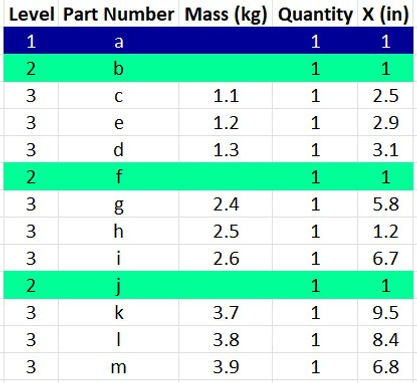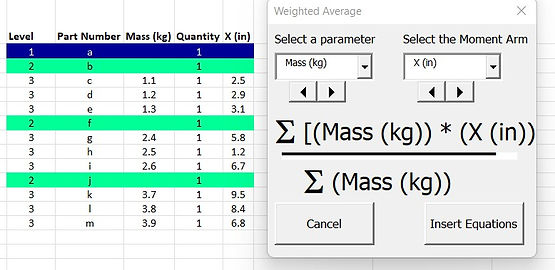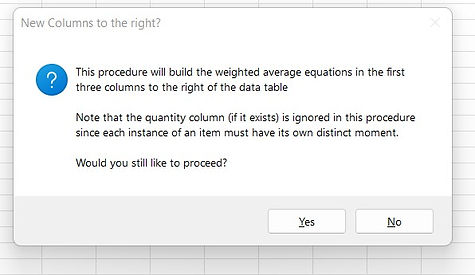top of page

# Weighted Averages

## There are a variety of contexts in which this function can be used, for instance, in calculating the center of gravity of a design.   Consider the table to the right, which has a 'Mass' column and the 'X (in)' column, representing the position of each item in the BOM along the 'X' axis in inches.## Once selected, the user can click on 'Insert Equations'## The message also notes that the 'Quantity' column, if it exists, is ignored, since each instance of an item must have it's own moment arm.## Equations are written in three columns appearing to the right of the table.  The first calculates and sums up the moments between the two columns.  The second has rollup equations.  The third column has the Weighted Average Calculations of the second parameter with respect to the first.bottom of page